Documentation

### This is machine translation

Mouseover text to see original. Click the button below to return to the English version of the page.

To view all translated materials including this page, select Country from the country navigator on the bottom of this page.

## Simulate the Physics of a Pendulum's Periodic Swing

This example simulates and explores the behavior of a simple pendulum by deriving its equation of motion, and solving the equation analytically for small angles and numerically for any angle.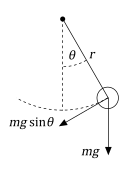1. Derive the Equation of Motion

2. Linearize the Equation of Motion

3. Solve Equation of Motion Analytically

4. Physical Significance of5. Plot Pendulum Motion

6. Understanding Non-Linear Pendulum Motion Using Constant Energy Paths

7. Solve Non-Linear Equation of Motion

8. Solve Equation of Motion for Closed Energy Contours

9. Solve Equation of Motion for Open Energy Contours

10. Conclusion

### 1. Derive the Equation of Motion

The pendulum is a simple mechanical system that follows a differential equation. The pendulum is at rest in a vertical position. We displace the pendulum by an angleand release it. The force of gravity pulls it back towards its resting position, its momentum causes it to overshoot and come to an angle(if there are no frictional forces), and so forth. The restoring force is, the gravitational force along the pendulum's motion (with a minus sign to remind us that it pulls back to the vertical position). Thus, according to Newton's second law, the mass times the acceleration must equal.

syms m a g theta(t) eqn = m*a == -m*g*sin(theta)
eqn(t) =The acceleration of the mass at the end of the pendulum isSubstitute for a by using subs.

syms r eqn = subs(eqn,a,r*diff(theta,2))
eqn(t) =Isolate the angular acceleration in eqn by using isolate.

eqn = isolate(eqn,diff(theta,2))
eqn =Collect constantsandinto a single parameter, called the natural frequency,syms omega_0 eqn = subs(eqn,g/r,omega_0^2)
eqn =### 2. Linearize the Equation of Motion

This equation is difficult to solve analytically because it is non-linear. Assuming the angles are small, we can linearize the equation by using the Taylor expansion of.

syms x approx = taylor(sin(x),x,'Order',2); approx = subs(approx,x,theta(t))
approx =The equation of motion becomes

eqnLinear = subs(eqn,sin(theta(t)),approx)
eqnLinear =### 3. Solve Equation of Motion Analytically

Solve the equation eqnLinear by using dsolve. Specify initial conditions as the second argument.

syms theta_0 theta_t0 theta_t = diff(theta); cond = [theta(0) == theta_0, theta_t(0) == theta_t0]; thetaSol(t) = dsolve(eqnLinear,cond)
thetaSol(t) =Simplify the solution by assumingis real using assume.

assume(omega_0,'real') thetaSol(t) = dsolve(eqnLinear,cond)
thetaSol(t) =### 4. Physical Significance ofis called the phase. The cosine functionrepeats every. The time needed to changebyis called the time periodFrom the equation, the time period is directly proportional to the pendulum's length. However,does not depend on the mass because its moment of inertia and its weight are both directly proportional to its mass.

The time period does not depend on the initial conditions but the amplitude does. Instead, the period is governed by the equation of motion.

### 5. Plot Pendulum Motion

Plot the motion of the pendulum for the small angle approximation.

Define physical parameters.

gValue = 9.81; % m / s^2 rValue = 1; % m omega_0Value = sqrt(gValue/rValue); t_0 = 2*pi/omega_0Value;

Set initial conditions.

theta_0Value = 0.1*pi; % Solution only valid for small angles. theta_t0Value = 0; % Initially at rest.

Substitute physical parameters and initial conditions into the general solution.

vars = [omega_0 theta_0 theta_t0]; values = [omega_0Value theta_0Value theta_t0Value]; thetaSolPlot = subs(thetaSol,vars,values); figure; fplot(thetaSolPlot(t*t_0)/pi, [0 1]);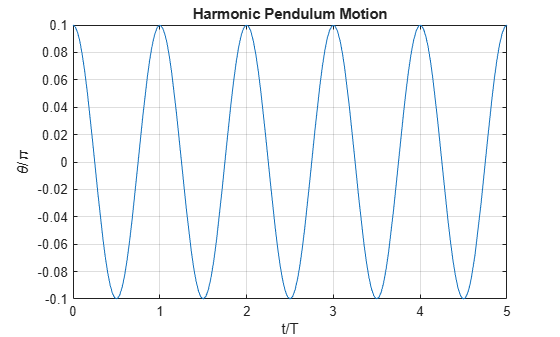fplot(thetaSolPlot(t*t_0)); grid on; title('Harmonic Pendulum Motion'); xlabel('t/t_0'); ylabel('\theta/\pi');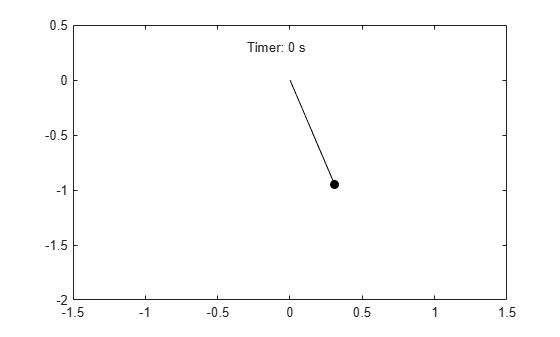Having found the solution for, we can visualize the motion of the pendulum below.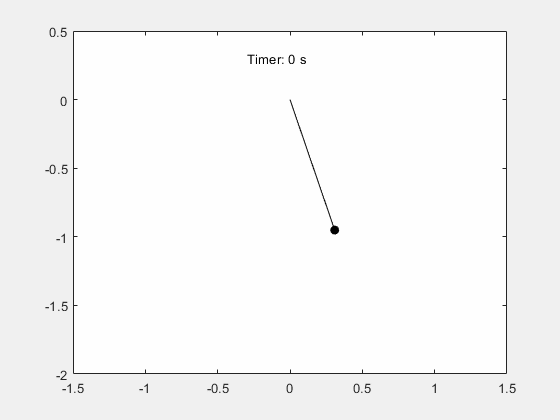### 6. Understanding Non-Linear Pendulum Motion Using Constant Energy Paths

To understand the non-linear motion of a pendulum, visualize the pendulum path by using the equation for total energy. Since the non-linear motion must conserve total energy, paths that have constant energy describe the non-linear motion.

The total energy isWe can use the trigonometric identityUse the relationto write the scaled energy asSince energy is conserved, the pendulum's motion can be described by constant energy paths in phase space, which is the abstract space with coordinatesvs.. We can visualize these paths using fcontour.

syms theta theta_t omega_0 E(theta, theta_t, omega_0) = (1/2)*(theta_t^2+(2*omega_0*sin(theta/2))^2); Eplot(theta, theta_t) = subs(E,omega_0,omega_0Value); figure; fc = fcontour(Eplot(pi*theta, 2*omega_0Value*theta_t), 2*[-1 1 -1 1], ... 'LineWidth', 2, 'LevelList', 0:5:50, 'MeshDensity', 1+2^8); grid on; title('Constant Energy Contours in Phase Space ( \theta vs. \theta_t )'); xlabel('\theta/\pi'); ylabel('\theta_t/2\omega_0');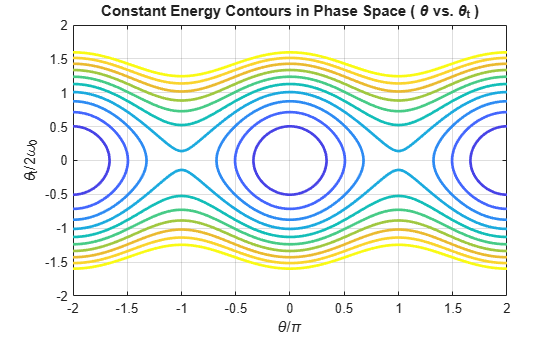The curves are symmetric about theaxis andaxis, and are periodic along theaxis. There are two regions of distinct behavior. The lower energies of the contour plot close upon themselves: the pendulum swings back and forth between two maximum angles and velocities.The higher energies of the contour plot do not close upon themselves because the pendulum always moves in one angular direction.### 7. Solve Non-Linear Equations of Motion

The non-linear equations of motion are a second-order differential equation. Numerically solve these equations by using the ode45 solver. Because ode45 only accepts first-order systems, reduce the system to a first-order system. Then, generate function handles that are the input to ode45.

Rewrite the second-order ODE as a system of first-order ODEs

syms theta(t) theta_t(t) omega_0 eqs = [diff(theta) == theta_t; diff(theta_t) == -omega_0^2*sin(theta)]
eqs(t) =eqs = subs(eqs,omega_0,omega_0Value); vars = [theta, theta_t];

Find the mass matrix M of the system and the right sides of the equations F.

[M,F] = massMatrixForm(eqs,vars)
M =F =M and F refer to the formTo simplify further computations, rewrite the system in the formf = M\F
f =Convert f to a MATLAB function handle by using odeFunction. The resulting function handle is input to the MATLAB ODE solver ode45.

f = odeFunction(f, vars)
f = function_handle with value: @(t,in2)[in2(2,:);sin(in2(1,:)).*(-9.81e2./1.0e2)] 

### 8. Solve Equation of Motion for Closed Energy Contours

Solve the ODE for the closed energy contours by using ode45.

From the energy contour plot, closed contours satisfy the condition,. Store the initial conditions ofandin the variable x0.

x0 = [0; 2*omega_0Value];

Choose a time interval that allows the pendulum to go through a full period. This can be found by trial and error.

tInit = 0; tFinal = 3.365*t_0;

Solve the ODE.

[t, x] = ode45(f, [tInit tFinal], x0);is returned in the first column of x, andis returned in the second column of x.

Scale the time dimension by the period,t = t/t_0;

Make the values ofrepeat on the intervalusing wrapToPi, then scaleby.

x(:,1) = wrapToPi(x(:,1))/pi;

Scaleby.

x(:,2) = x(:,2)/(2*omega_0Value);

Plot the closed path solution.

figure; yyaxis left; plot(t, x(:,1), '-o'); ylabel('\theta/\pi'); yyaxis right; plot(t, x(:,2), '-o'); ylabel('\theta_t/2\omega_0'); grid on; title('Closed Path in Phase Space'); xlabel('t/t_0');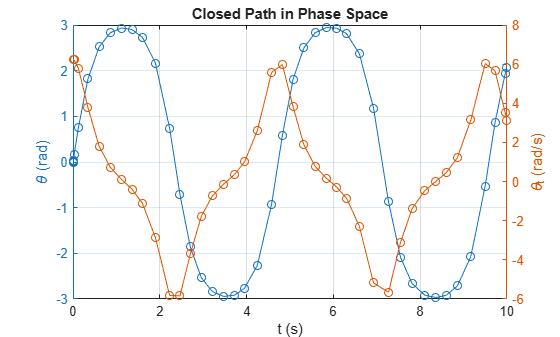This is how the pendulum motion would appear:### 9. Solutions on Open Energy Contours

Solve the ODE for the open energy contours by using ode45.

From the energy contour plot, open contours satisfy the condition,.

x0 = [0; 1.001*2*omega_0Value]; tFinal = 1.465*t_0; [t, x] = ode45(f, [tInit, tFinal], x0); t = t/t_0; x(:,1) = wrapToPi(x(:,1))/pi; x(:,2) = x(:,2)/(2*omega_0Value); figure; yyaxis left; plot(t, x(:,1), '-o'); ylabel('\theta/\pi'); yyaxis right; plot(t, x(:,2), '-o'); ylabel('\theta_t/2\omega_0'); grid on; title('Open Path in Phase Space'); xlabel('t/t_0');This is how the pendulum motion would appear:### 10. Conclusion

We have derived the simple pendulum's equation of motion, linearized and solved its equation of motion analytically, visualized its energy contours to understand the system qualitatively, and solved the general equation of motion numerically for particular initial conditions.

### Helper Functions

function lambda = wrapToPi(lambda) %wrapToPi Wrap angle in radians to [-pi pi] % % lambdaWrapped = wrapToPi(LAMBDA) wraps angles in LAMBDA, in radians, % to the interval [-pi pi] such that pi maps to pi and -pi maps to % -pi. (In general, odd, positive multiples of pi map to pi and odd, % negative multiples of pi map to -pi.) q = (lambda < -pi) | (pi < lambda); lambda(q) = wrapTo2Pi(lambda(q) + pi) - pi; end function lambda = wrapTo2Pi(lambda) %wrapTo2Pi Wrap angle in radians to [0 2*pi] % % lambdaWrapped = wrapTo2Pi(LAMBDA) wraps angles in LAMBDA, in radians, % to the interval [0 2*pi] such that zero maps to zero and 2*pi maps % to 2*pi. (In general, positive multiples of 2*pi map to 2*pi and % negative multiples of 2*pi map to zero.) positiveInput = (lambda > 0); lambda = mod(lambda, 2*pi); lambda((lambda == 0) & positiveInput) = 2*pi; end

#### Mathematical Modeling with Symbolic Math Toolbox

Get examples and videos